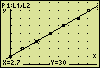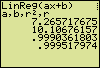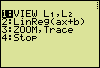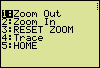# Linear Regressiona program for the Sharp EL-9600, EL-9650and EL-9900 graphing calculators

### Summary

This program is a shortened version of the program Regression in which more regression models are integrated. Regression has been written for the TI-83 Plus calculator. It should run as well on a TI-83 and more recent models.

Every measurement is influenced by many uncertainties. Measurement uncertainties can never be completely eliminated, so the true value for any quantity is never known exactly.
If two physical quantities are known to have a linear relationship, experimental data, when plotted on a graph, are expected to fit a straight line in a more or less accurate and precise way. Due to errors in the measuring process, not all the data fall exactly on the drawn line.(TI images. The screen examples
slightly differ from Sharp pictures)

Linear regression is a statistical tool that helps you to determine the "best-fitting" straight line for any given set of experimental data. In the relationship  y = ax + b  the constants a and b are being determined. The obtained function hands over which value is to be expected on an average for the one variable (y) at a given value of the other variable (x).You might prefer knowing more about linear regression beforehand. If so, it is recommended to view an interactive demonstration in order to understand the basic outcomes a linear regression analysis has to offer.

### Program Listing

Help
PROGRAM:REGLIN R : ALPHA, R keys
ClrT > 2ndF, PRGM > SCRN > ClrT
Rect:RectCursor > 2ndF, PRGM > COORD > Rect
: : ALPHA, : keys
ExprOn:Connect > 2ndF, PRGM > FORM > Connect
DrawOFF > 2ndF, DRAW > ON/OFF > DrawOFF
Print "DO BEFOREHAND:" > 2ndF, PRGM > PRGM > Print
> 2ndF, PRGM > PRGM > "
Space : ALPHA, .. (above . key)
Print "1. PLOTOFF" important reminders
Print "2. STORE VALUES"
Print "INTO L1 AND L2." L1 : 2ndF, L1 keys
Wait > 2ndF, PRGM > PRGM > Wait
Plt1(Scattr§,L1,L2) > 2ndF, PRGM > S_PLOT > Plt1(
> 2ndF, STAT PLOT > S.D. > Scattr§ (§ : small square)
Zm_Stat > ZOOM > ZOOM > Stat
(max(L1)–min(L1))/5 → U > MATH > NUM > max(
"→" Symbolizes the STO key
10^(int (log (U))) → Z 10^( : 2ndF, 10x keys
> MATH > NUM > int
U/Z → U
(int (√(U–1))²+1)Z → Xscl √ : 2ndF, √ keys (square root)
² : x² key
> VARS > WINDOW > ENTER > XY > Xscl
(max(L2)–min(L2))/5 → V
10^(int (log (V))) → Z
V/Z → V
(int(√(V–1))²+1)Z → Yscl (square root)
StoWin > ZOOM > STO > StoWin
Label 0 > 2ndF, PRGM > BRNCH > Label
0 → N : ClrT
Print "1 VIEW L1,L2 (END) , : comma key
Print "2 Rg_ax+b
Print "3 ZOOM,TRACE > TRACE
Print "4 STOP
Print "
Print "NUMBER 1-4
Input N > 2ndF, PRGM > PRGM > Input
ClrT
If N=1 Goto 1 > 2ndF, PRGM > BRNCH > If (Goto)
If N=2 Goto 2
If N=3 Goto 60
Xscl → TBLStep > VARS > TABLE > TBLStep
End > 2ndF, PRGM > PRGM > End
Label 60
Print "1 Zm_Out > ZOOM > ZOOM > Out
Print "2 Zm_In
Print "3 RESET ZOOM
Print "4 TRACE
Print "5 HOME
Print "
Input N
ClrT
If N=1 Goto 61
If N=2 Goto 62
If N=3 Goto 63
If N=4 Goto 64
Goto 0
Label 1
Print L1:Wait :ClrT
Print L2:Wait
Goto 0
Label 2
Print "Rg_ax+b " > STAT > REG > Rg_ax+b
Print "a,b,r²,r" > VARS > STAT > ENTER > REGEQN > a (and b,r²,r)
Rg_ax+b (L1,L2,Y1) > VARS > EQVARS > ENTER > XY > Y1
Print a
Print b
Print r²
Print r
Wait
DispG > 2ndF, PRGM > SCRN > DispG
Wait
Goto 0
Label 61
√2 → Z : Z → X_Fact (square root)
Z → Y_Fact > VARS > WINDOW > XY > X_fact
10^(int (log ((Xmax–Xmin)/Z))) → Xscl > VARS > WINDOW > XY > Xmax
10^(int (log ((Ymax–Ymin)/Z))) → Yscl
Zm_Out > ZOOM > ZOOM > Out
Wait
Goto 60
Label 62
Zm_In:Wait
Goto 60
Label 63
RclWin:Wait > ZOOM > RCL > RclWin
Goto 60
Label 64
TRACE > TRACE
Goto 60

### An Application

After putting in the program listing, you might like to see the program working.
Before starting the program, possible active statistical plots have to be disabled with the "PlotOFF" command.
PlotOFF is not programmable. At the normal screen press:
> 2ndF, STAT PLOT > ON/OFF > PlotOFF.
Having done so, try the following example.

### The Experiment

A long tube is placed vertically and filled with a viscous liquid. (Which liquid is not?) When a bullet, being made up of steel, is let loose in the liquid, the sinking speed soon becomes constant. To determine this constant velocity, the position x is measured as a function of time t. The measuring outcome is:

 t(s) x(cm) 0,01,32,74,25,57,08,1 10203040506070

### Entering the Values

The time t is considered the independent variable and is measured along the horizontal axis of a graph. The position x, the dependent variable, is measured along the vertical axis. List 1 (L1) must contain values of the variable, which is to be plotted along the horizontal axis.
So, store the time values in List 1 and the position values in List 2 (L2). Here's how you can get access to these lists: > STAT > EDIT > ENTER > ...(TI images. The screen examples
differ slightly from Sharp pictures)

### Getting the Linear Equation and the Coefficient of Correlation

After preparing L1 and L2, run the program. A menu appears.
Select option "2 Rg_ax+b" by pressing 2 and ENTER.
A fysical equivalent to the shown equation  y = ax + b  is the position equation
x(t) = v t  +  x(0).
The displayed value of the constant a in  y = ax + b  equals 7.26571..., meaning the constant velocity v amounts to 7,27 cm/s.
The position at the beginning of the measurements is
x(0) = = 10,1 cm.
Substituting the numerical values in the position equation leads to the linear equation in demand:
x(t) = 7,27 t  +  10,1  with x expressed in cm.The produced value of the coefficient of correlation (r = 0,99952) indicates that the found linear equation is only a fair representation of the relationship between the measured t and x values. Counting the nines, that directly follow the comma in the coefficient of correlation, is a convenient way to find out the usefulness of the equation. In chemical and physical determinations the number of nines may be interpreted in this way: five nines mean an excellent representation, four nines a good one, three nines a fair one, two nines a poor one, and one nine means a very poor representation. With no nines directly behind the comma the equation is "unusable".

### Viewing the Plotted Points and the Straight Line

Press ENTER.### Tracing

Once the graph has been drawn, press ENTER, select option "3 ZOOM,TRACE" in the menu, press ENTER, select "4 TRACE" in the following sub-menu and press ENTER.
With the up arrow key, choose the plotted measuring points, and use the left and right arrow keys to trace the values of these points.Press the up arrow key once to enable tracing the plotted line. Again, use the left and right arrow keys to explore the coordinates of some points of the line.Using the TABLE function is another way to get values of points of the line. Stop the execution of the program by selecting "5:HOME" in the sub-menu and "4 STOP" in the main menu. Then call on the TABLE function. You may have to adjust the table settings using the TBLSET function.Hein ten Horn
Top | Programs Sharp EL | Home